Welcome to Exploring similar triangles! Learning about similar triangles is not only an important math skill, but it also has many practical applications. Your real-life knowledge of similar triangles is used every day when you consider architecture, bridges, the length of shadows, or the correct angle to swing a baseball bat.

Many students try to be successful in high school mathematics by memorizing, memorizing, memorizing. There are some things you do need to memorize, such as the times tables, but reflecting on your learning and making connections between topics will take you much further. Let’s get started!

### Math journal

Utilize any journal, notebook, or logging document of your choice as a math journal, which will help you reflect on your learning during this course.

You will be building your math journal throughout this course which consists of 4 units:

• Similar triangles, right-angled trigonometry and three-dimensional measurement
• Linear relations and systems
• The algebra of quadratic expressions#### Math journal tips

At the end of each learning activity you will be prompted to create a journal entry, with suggestions and guidelines about the content. These journal entries will serve as a summary of many of the important concepts in this course and will be useful when you are preparing for the unit assessments and the final exam. As such, they should be written in a way that will be most meaningful to you.

Please feel free to add any pieces of information that you think are important in addition to the suggested content. For your first journal entry, take a few minutes to research an interesting fact on how similar triangles are applicable to everyday life! Starting any learning activity with curiosity can motivate and make the learning process enjoyable and purposeful!

At the end of the course, you will have the opportunity to fine-tune 8 entries from your math journal and submit them as a culminating assessment. They will be worth 15% of your grade.This image is a visual reminder that you should be adding to your math journal.

At the beginning of unit 2, you will have the oppportunity to submit one journal entry for feedback. You may choose to make any suggested edits based on that feedback and include the entry in your culminating assessment. This is an opportunity to improve your journal writing success!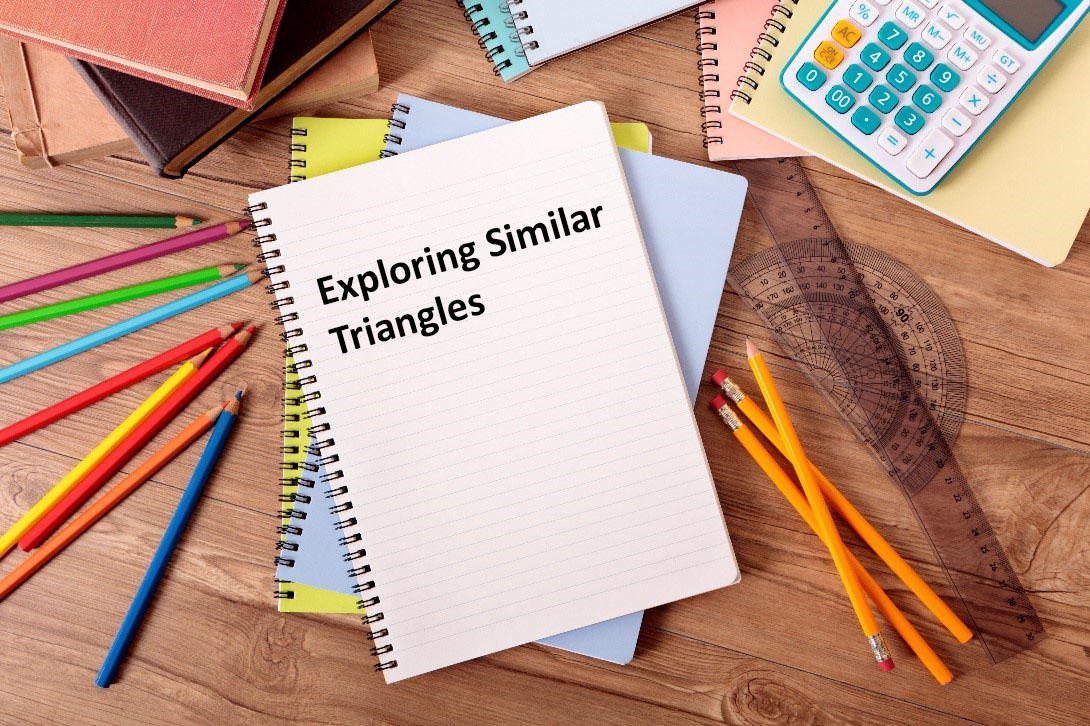Each of the 8 journal entries that you submit should include:

• the journal entry title and description, including the Unit and Learning Activity numbers
• evidence of learning from that activity (this could include picture(s) of worked examples, written explanations, a summary sheet, etc.)
• evidence of mathematical thinking, as demonstrated by an example of a ‘real world application problem, including its solution, that is connected to the learning, or a model that explains the mathematical concept being applied. In the sample journal entries this would be observed in the fraction diagram that explains equivalent fractions or the use of geometry software to measure the railing angle. In both sample entries, real world problems are used to demonstrate thinking.
• Correct use of mathematical conventions
• A conclusion or statement to demonstrate reflection.

Your math journal entries can be presented in a variety of ways. Here are some suggestions

• handwritten journal (scanned)
• online journal
• video recordings
• pictures
• audio recordings
• mind map

### Rubric

Please read through the rubric below to ensure full understanding of the assessment guidelines.

#### Knowledge and Understanding [5 marks]

Success Criteria:

• Demonstrates knowledge of relevant and appropriate skills and procedures
• Demonstrates understanding of the meaning of the mathematical content
 Level 4 (80 – 100%) Level 3 (70 – 79%) Level 2 (60 – 69%) Level 1 (50 – 59%) With a high degree of effectiveness With considerable effectiveness With some effectiveness With limited effectiveness

#### Thinking [5 marks]

Success Criteria:

• Shows evidence of modelling the problem, drawing conclusions, or justifying reasoning
• Demonstrates a logical interpretation of problem
 Level 4 (80 – 100%) Level 3 (70 – 79%) Level 2 (60 – 69%) Level 1 (50 – 59%) With a high degree of effectiveness With considerable effectiveness With some effectiveness With limited effectiveness

#### Application [5 marks]

Success Criteria:

• Illustrates relevant and appropriate selection of facts, skills and procedures
• Demonstrates relevant and appropriate connections made between math concepts and the world outside the classroom
 Level 4 (80 – 100%) Level 3 (70 – 79%) Level 2 (60 – 69%) Level 1 (50 – 59%) With a high degree of effectiveness With considerable effectiveness With some effectiveness With limited effectiveness

#### Communication [5 marks]

Success Criteria:

• Uses math vocabulary, notation and symbols
• Writes organized algebraic solutions, graphs, charts and diagrams clearly
• Expresses a clear reflection of mathematical thinking
 Level 4 (80 – 100%) Level 3 (70 – 79%) Level 2 (60 – 69%) Level 1 (50 – 59%) With a high degree of effectiveness With considerable effectiveness With some effectiveness With limited effectiveness

Here is sample math journal entry document(Opens in a new window) to help you approach your journal entries!

## Similar Figures

Similar figures occur very often in the world around us. Examine the three images of the same boat. The images are the same shape, so they are similar figures. We will get into a more specific definition in a bit.  The biggest boat picture is twice as long and twice as high as the bottom images. A photo enlargement will always create a similar figure to the original photo.If we compare all three pictures, even though one is the reverse image of the other, they are all similar figures.  Similar figures can also be the same size; they don’t have to be larger than the others.

## Similar Triangles

### Definition:

Similar triangles are triangles that are the same shape. There are two geometric relationships (between angles and sides) that make them similar. Below we will find two triangles that illustrate the concept of similarity between triangles. Please note that the angles and sides listed in the diagrams are for explanation purposes only. Triangles don’t have to have 30°, 45° and 105° angles to be similar or have these specific side lengths.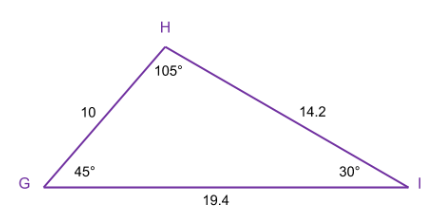ΔABC ~ ΔGHI is how you write mathematically that ΔABC is similar to ΔGHI. This is called the similarity relationship.

Similar triangles are the same shape. This means that every angle in one triangle has an equal corresponding angle in the second triangle.

Equal angle pairs:

∠ A = ∠ G

∠ B = ∠ H

∠ C = ∠ I

You will often use ratios of sides to show triangles are similar, but before we get into that, a little more about angles.

If two triangles have two pairs of equal angles, then the third in each must also be the same (since the three angles always add to 180°).

So, as soon as you see two triangles with two equal pairs of angles, then the triangles must be similar. This justification is often called Angle-Angle Similarity, or simply, AA. You can use the acronym AA when explaining why two triangles are similar, assuming the triangles have two pairs of equal angles.

The ratios of the corresponding sides are constant. This means that if we divide a side length from one triangle by its corresponding side in the other triangle, that ratio will be the same for all three pairs of sides.

### Relationship between sides:

In

$\angle A=\angle G$ . The side across from $\angle A$ is $BC$. The side across from $\angle G$ is $HI$. So, $BC$ is said to correspond to $HI$. In the same way, $\angle B=\angle H$, so $AC$ corresponds to $GI$ and since $\angle C=\angle I$ then $AB$ corresponds to $GH$.

Let’s look at the ratios of the corresponding sides.

Since all three ratios equal $\mathrm{½}$we write $\frac{AB}{GH}$= $\frac{BC}{HI}$ = $\frac{AC}{GI}$

These are the ratios of the corresponding sides.

For example, if $GH=2×AB$, the $HI=2×BC$ and $GI=2×AC$

It doesn’t matter which triangle has the sides on the top or bottom of these ratios. We could have written the following instead: $\frac{GH}{AB}$ = $\frac{HI}{BC}$ = $\frac{GI}{AC}$ Since the larger sides are in the numerator, the ratio would be $\frac{2}{1}$ or

The order the similarity statement is written is important.In the similarity statement above $A$ and $B$ are first & second, so are $G$ and $H$ so $AB$ corresponds to $GH$.

In the similarity statement above $B$ and $C$ are second & third, so are $H$ and $I$ so $BC$ corresponds to $HI$.

In the similarity statement above $A$ and $C$ are first & third, so are $G$ and $I$ so $AC$ corresponds to $GI$.

### Relationship between sides:

Or

Explore these similar triangles by changing the side lengths and angles. Put your curser on C or B in the first triangle. The two things you should pay close attention to are the matching angles and the ratios of the corresponding sides.

This is an assessment for learning (not for marks):Use the diagram representing $\Delta BCD$ and $\Delta FMJ$, to answer the following questions.Throughout the course you should make a conscious effort to use the formula sheetOpens in a new window that you will be made available to you when you write your final exam.Here are a few practice exercises you can try. Solutions are included for each question.

State if the following pairs of triangles are similar and explain your reasoning. Solutions are available.State if the following pairs of triangles are similar and explain your reasoning. Solutions are available.State if the following pairs of triangles are similar and explain your reasoning. Solutions are available.State if the following pairs of triangles are similar and explain your reasoning. Solutions are available.Given the diagram: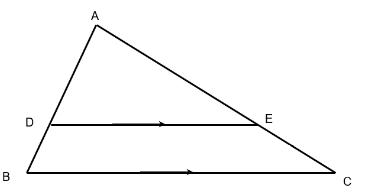Write the similarity relationship for these triangles.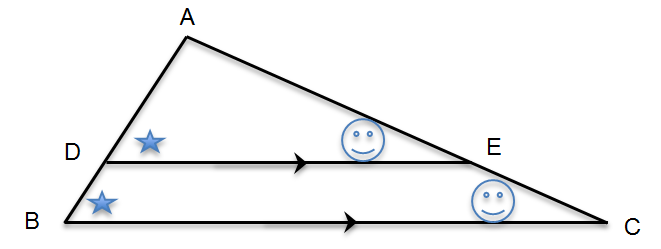Sometimes this is easier to visualize if you redraw with two separate triangles like this:

These triangles are similar by AA.

Note: Another reason ∠DAE = ∠BAC and ∠ADE = ∠ACB is called the F Pattern from the grade 9 course.

The pair of angles labelled with the stars correspond (and are equal) and the pair labelled with the happy faces are also corresponding (and equal as well).

Given the diagram:List the equal pairs of angles for these similar triangles.

Given the diagram:Write the ratios of sides for these similar triangles.

You are told that ΔBCO ~ ΔMRP.

Draw a diagram (there are many correct ways to do this) that shows this similarity relationship.

Determine whether the pair of triangles are similar (or not). Explain your reasoning.Determine whether the pair of triangles are similar (or not). Explain your reasoning.For the pair of similar trianglesState the similarity relationship

For the pair of similar triangles, state the pairs of equal anglesFor the pair of similar triangles, state the ratios of sidesFor the pair of similar triangles, state the similarity relationship.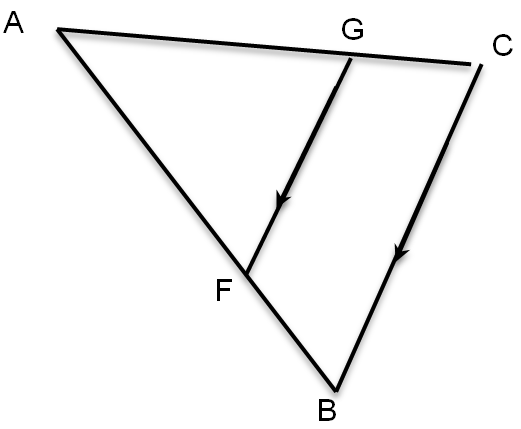For the pair of similar triangles State the pairs of equal anglesFor the pair of similar triangles, state the ratios of sidesCongratulations! You have now completed Learning Activity 1. By working through all of the examples… you’ll likely feel very comfortable with how to:

• write the similarity relationship between two triangles
• determine when triangles are similar (or not)

Take some time to reflect on this learning activity.

Which level of comfort below is most reflective of your learning? Don’t worry, you can master the concepts with revisiting examples and more practice!

Agree or Disagree statements
Success Criteria I feel I have mastered the concepts I feel close to mastering the concepts I feel I still need to go over additional examples to master the concepts I’m not quite there yet in mastering the concepts
write the similarity relationship between two triangles
determine when triangles are similar (or not)

As a self-directed learner, you will be reflecting on your learning process and checking your understanding in order to plan for success.

Think about how comfortable you are with the success criteria listed below. Is something still unclear? If so, take some time and review the relevant parts of the learning activity, or go online and do additional research.

Don’t forget about Mathify (Opens in new window). It’s open Sunday through Thursday from 3:30 to 9:30 p.m. Eastern Time (except for holidays).

Disclaimer: Mathify is for learners in Ontario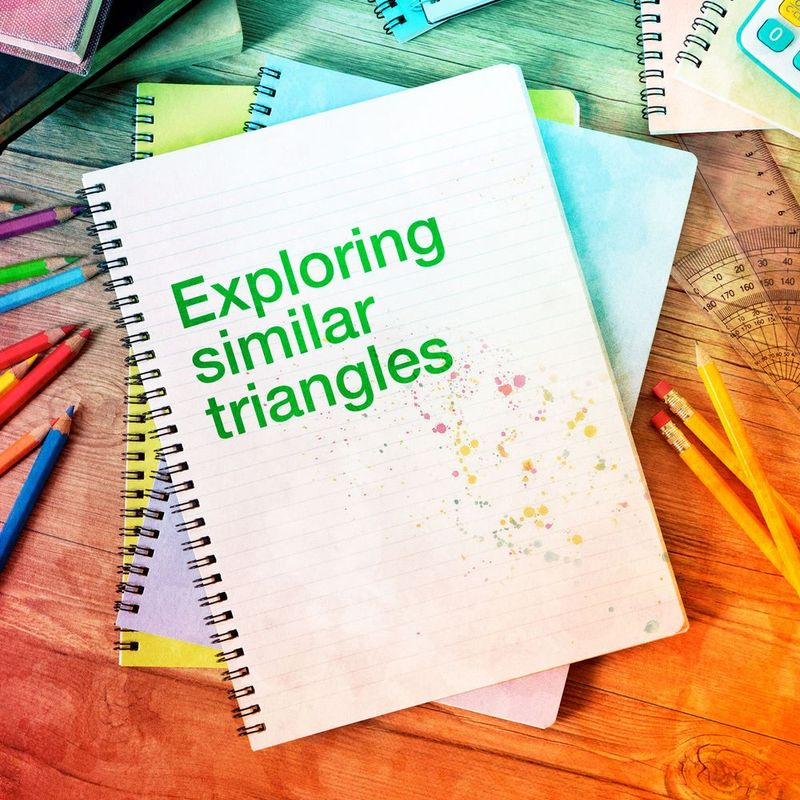Remember that, at the end of the course, you will fine-tune 8 entries from your math journal and submit them as your “Culminating assessment - Math journal”

Check the requirements for journal entries to make sure you include all the important elements of this task. They will be worth 15% of your grade.

Always refer to the success criteria when selecting a topic for your journal entry. For this learning activity, you could write about how to determine when two triangles are similar.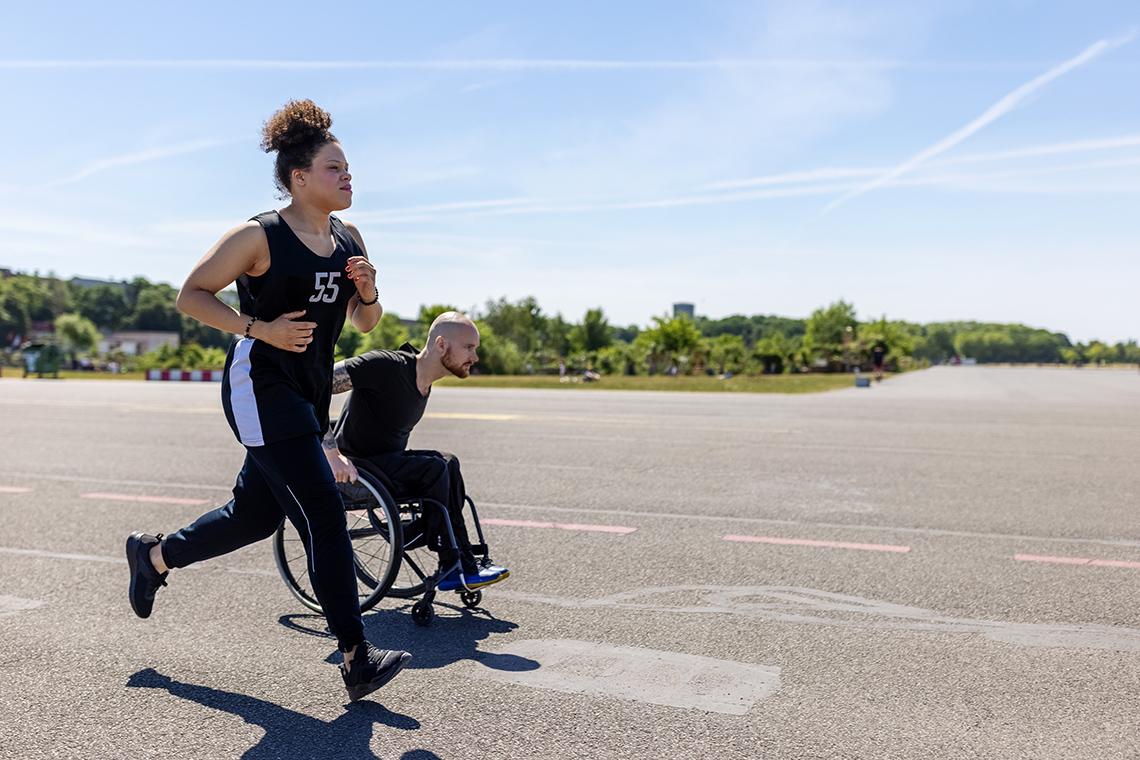Let’s get energized! In Learning activity 2, we will develop an important skill set: Problem solving using similar triangles. This will help you learn how to apply the theory relating to triangles and find solutions to everyday problems.